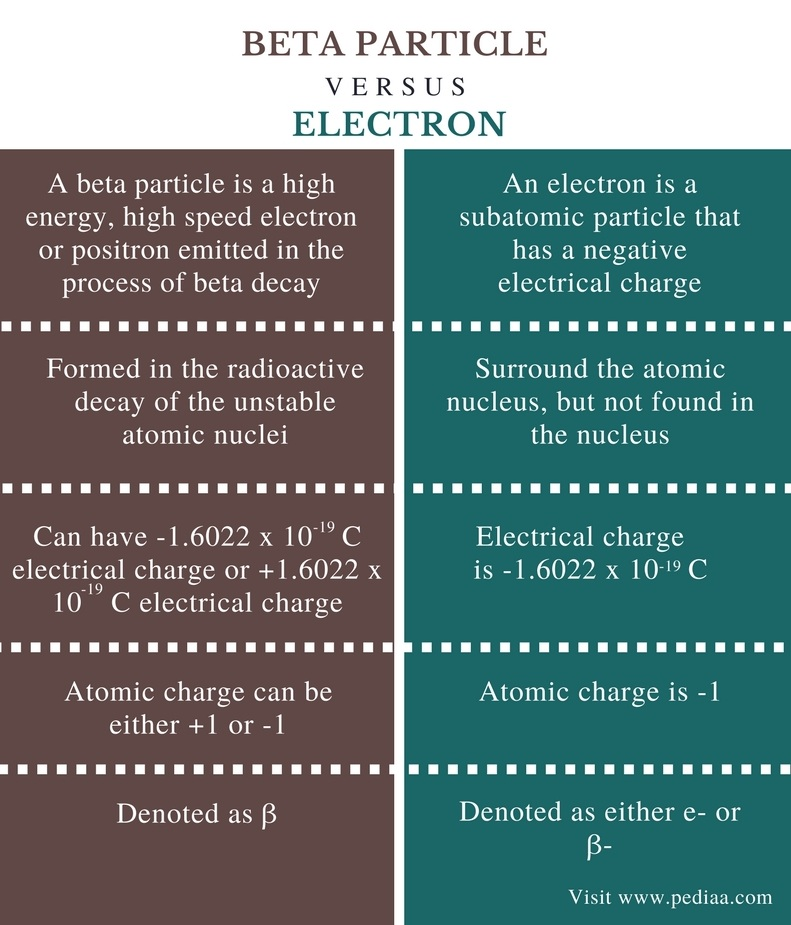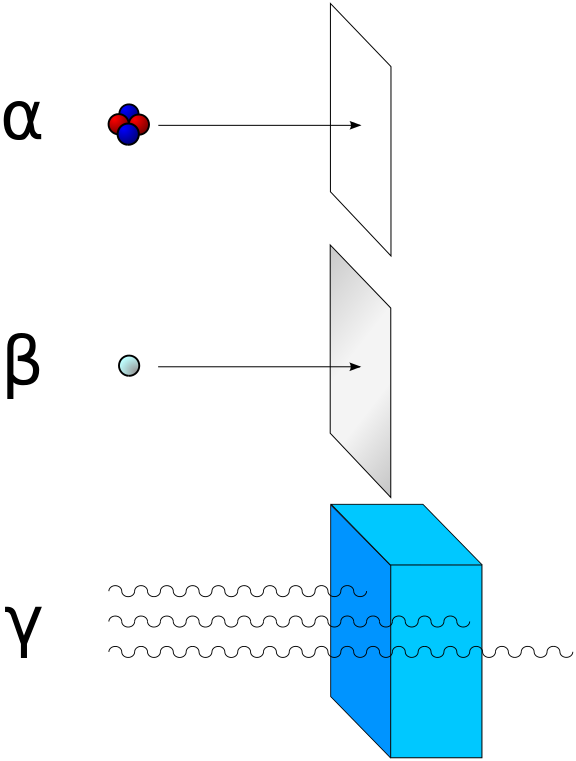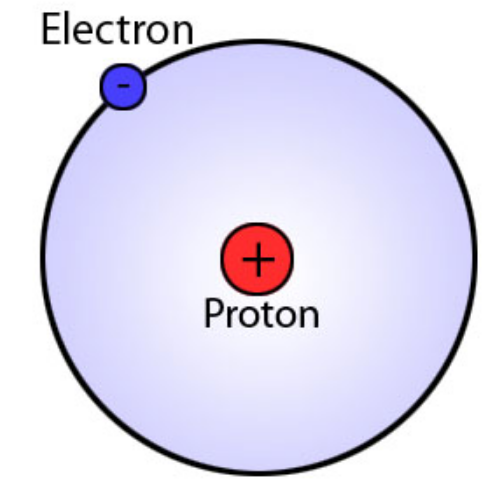# Difference Between Beta Particle and Electron

## Main Difference – Beta Particle vs Electron

Beta particles are the subatomic particles that are emitted during beta decay. Beta particles can be either electrons or positrons. If it is an electron, that beta particle has a negative electrical charge, but if it is a positron, it has a positive electrical charge. Electrons are subatomic particles that can be found in the electron cloud that surrounds the atomic nucleus. The main difference between beta particle and electron is that beta particle can have either +1 charge or -1 charge whereas electron has a -1 charge.

### Key Areas Covered

1. What is a Beta Particle
– Definition, Explanation, Uses
2. What is an Electron
– Definition, Properties
3. What is the Difference Between Beta Particle and Electron
– Comparison of Key Differences

Key Terms: Atom, Atomic Nucleus, Beta Decay, Beta Particle, Electron, Gamma Ray, Neutron, Probability, Proton, Radioactive## What is a Beta Particle

A beta particle is a high energy, high speed electron or positron emitted in the process of beta decay. It is denoted by the symbol “β”. Beta particles are emitted during the radioactive decay of an unstable atomic nucleus. There are two types of beta particles as β+ particle or positron and β particle or electron.

β decay is also known as electron emission since β particle is an electron. This type of radioactive decay occurs in unstable nuclei with an excess of neutrons. Here, the conversion of a neutron into a proton and an electron occurs. This type of decay does not change the atomic mass, but the atomic number is changed.

Β+ decay is also known as positron emission since a β+ particle is a positron. This type of decay occurs in atomic nuclei having an excess of protons. Here, a proton is converted into a neutron and a positron. This type of decay causes the change of atomic number but no the atomic mass.

Beta radiation is a type of ionizing radiation. Beta particles have a moderate penetration strength when beta radiation is directed to a substance. Ionization strength is also medium to that of alpha rays and gamma rays. The ionizing energy of beta ray occurs due to the presence of charged particles (electron are negatively charged; positrons are positively charged).Figure 1: Ionizing Power of Beta Ray is Moderate when Compared to Alpha Ray and Gamma Ray

Beta particles have medicinal applications. Beta particles are used to treat eye cancers and bone cancers. In addition, beta particles or beta radiation is used to determine the thickness of a substance such as paper. Positron decay of a radioactive tracer isotope is the source of positrons used in PET (positron emission topography).

## What is an Electron

Electron is a subatomic particle that has a negative electrical charge. Electrons are known to be located in the electron cloud that surrounds the atomic nucleus, and these particles are in movement in specific pathways known as electron shells. There is a high probability of finding an electron near the atomic nucleus. However, there are no electrons in the atomic nucleus. The electron is denoted by e or β.

The electrical charge of an electron is -1.6022 x 10-19 C and the mass of an electron is 9.1094 x 10-28 g. The mass of electron is negligible when compared to the mass of a proton and a neutron (mass of both particles is 1.6740 x 10-24 g; hence the mass of an electron is only 1/1,836 the mass of a proton.). But the atomic charge of an electron is given as -1 and the atomic mass as 0.00054858 amu.Figure 2: There are no Electrons in the Atomic Nucleus

The electron was discovered by Sir J.J. Thomson. According to the standard model of particle physics, electrons belong to the group of subatomic particles known as leptons. Leptons are believed to be the elementary particles. Electrons have the lowest mass among other lepton particles

## Difference Between Beta Particle and Electron

### Definition

Beta Particle: A beta particle is a high energy, high speed electron or positron emitted in the process of beta decay.

Electron: An electron is a subatomic particle that has a negative electrical charge.

### Origin

Beta Particle: Beta particles are formed in the radioactive decay of the unstable atomic nuclei.

Electron: Electrons are already in the atom surrounding the atomic nucleus, no electrons can be found in the nucleus.

### Electrical Charge

Beta Particle: A beta particle can have either -1.6022 x 10-19 C electrical charge or +1.6022 x 10-19 C electrical charge.

Electron: The electrical charge of an electron is -1.6022 x 10-19 C.

### Atomic Charge

Beta Particle: The atomic charge of a beta particle can be either +1 or -1.

Electron: The atomic charge of an electron is -1.

### Denotation

Beta Particle: A beta particle is denoted as β (it can be either β+ or β).

Electron: An electron is denoted as either e or β.

### Conclusion

Beta particles can be either electrons or positrons. These particles are originated from atomic nuclei during the beta decay. Electrons are already in the atoms surrounding the atomic nucleus (in electron cloud). The main difference between beta particle and electron is that beta particle can have either +1 charge or -1 charge whereas electron has a -1 charge.

##### Reference:

1. “Beta particle.” Wikipedia, Wikimedia Foundation, 31 Jan. 2018, Available here.
2. “Sub-Atomic Particles.” Chemistry LibreTexts, Libretexts, 21 July 2016, Available here.
3. “Electron.” Encyclopædia Britannica, Encyclopædia Britannica, inc., 2 Nov. 2017, Available here.

##### Image Courtesy:

1. “Alfa beta gamma radiation” By User:Stannered – Traced from this PNG image (CC BY 2.5) via Commons Wikimedia
2. “Bohr model” By Jia.liu – Own work (Public Domain) via Commons Wikimedia